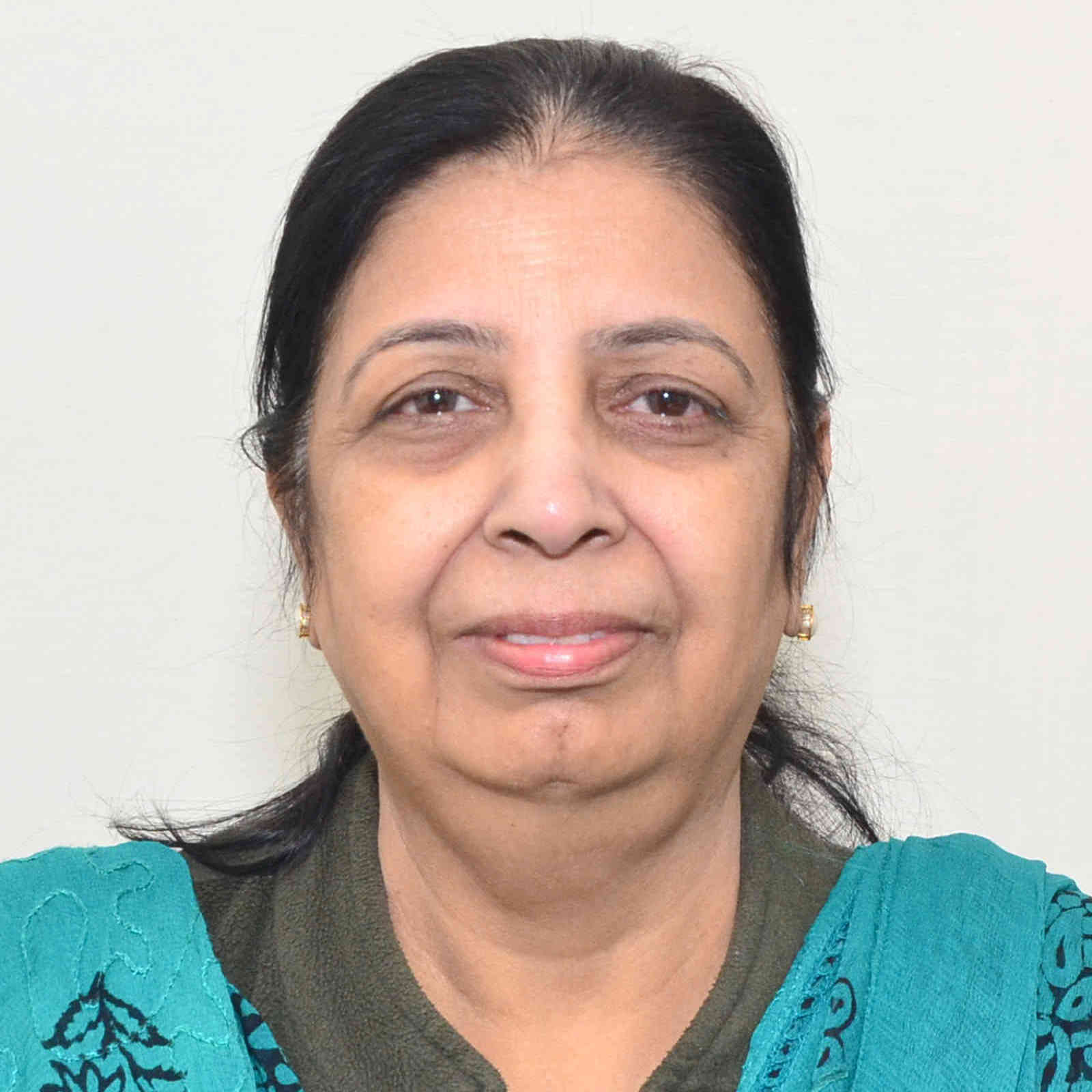Faculty List - Department of Mathematics,Indian Institute of Technology Roorkee
Faculty List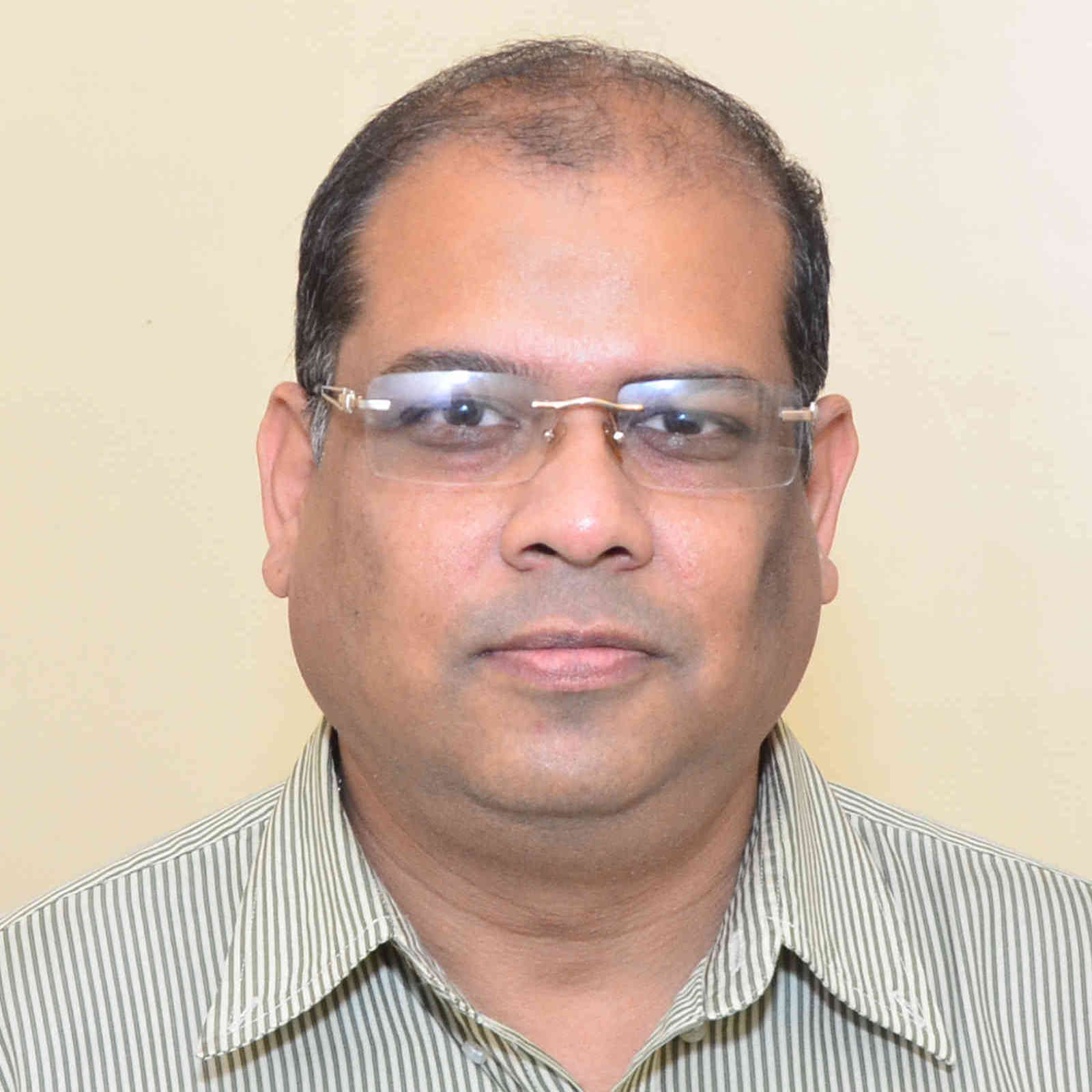Professor
Interests :

Mathematical Biology, Mathematical Modeling.,

sandip.banerjee@ma.iitr.ac.in(01332)285697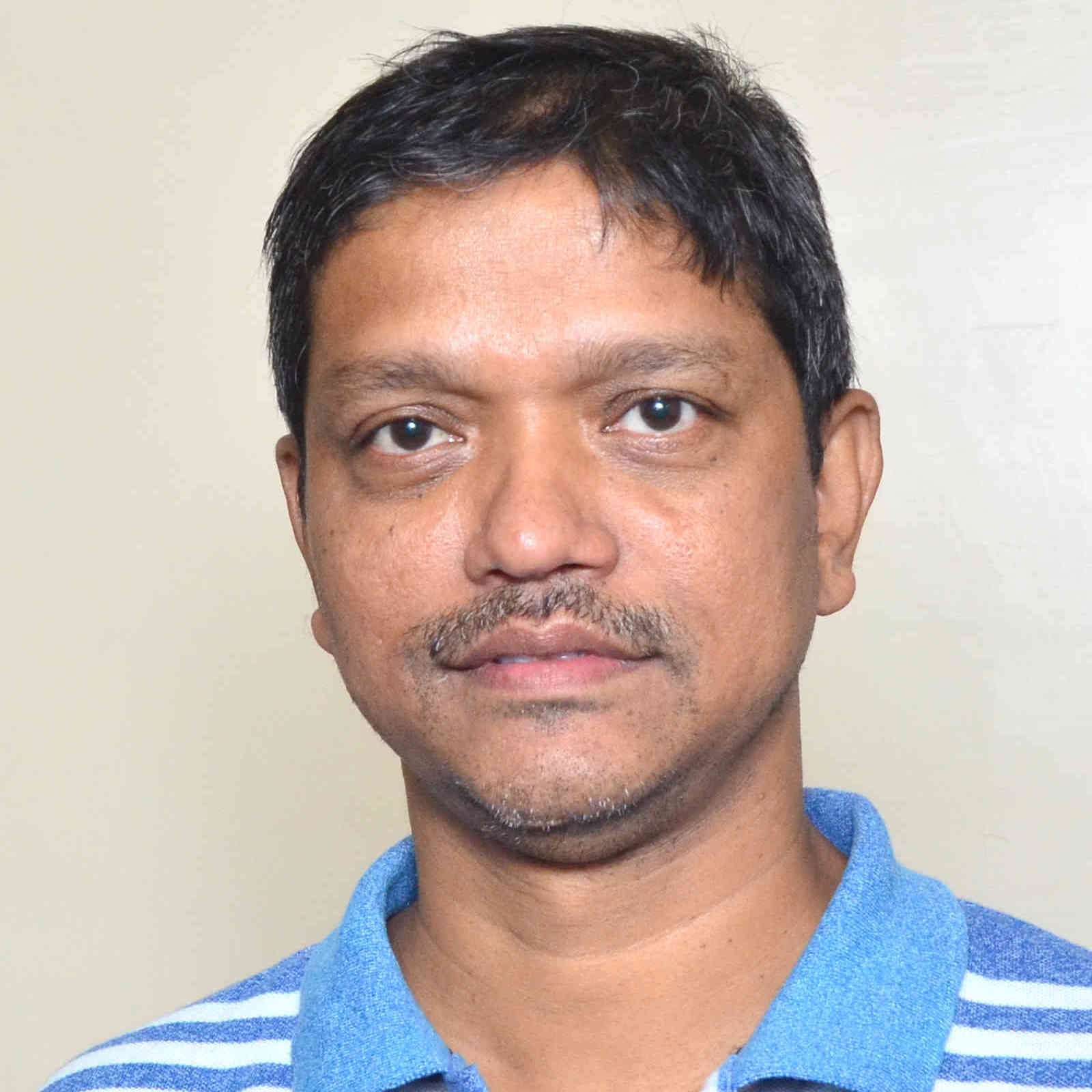Interests :

Mechanisms of miscible fluid flow in porous medium, Convection in porous media, Hydrodynamic stability,, Non-conforming spectral element methods: theory and applications, Hydrodynamics using Spectral methods / Spectral element methods / finite difference methods,,

p.bera@ma.iitr.ac.in01332-285071Professor
Interests :

Operations Research, Design of Nature Inspired Optimization Techniques, Soft Computing , Computational Intelligence, Neural Networks, Fuzzy, Numerical Optimization, Evolutionary Algorithms, Swarm Intelligence, Parallel Computing , Parallel Nature Inspired Optimization, Membrane Computing, P-Systems, GPU computing, Applications, Forecasting of Landslides, Avalanches, Earthquake, Finance, Applications, Games like Sudoku, Chess,

kusum.deep@ma.iitr.ac.in09837164078Associate Professor
Interests :

Statistics and related areas, Data analytics, Discrete Probability, Cryptography, Financial Mathematics, Statistical Inference.,Assistant Professor
Interests :

Algebraic Topology, Equivariant homotopy theory,

surojit.ghosh@ma.iitr.ac.inAssociate Professor
Interests :

Partial Integro-Differential Equations, Coagulation-Fragmenation Models: Well-posedness, Regularity, Mass-conservation, Gelation, Large time behaviour, Stationary solutions, Self-similar profiles, Numerical Methods, Error Analysis, Stochastic Partial Differential Equations: Well-posedness, Regularity and Convergence Analysis of Numerical Methods, Regularization Theory for Inverse Problems: Variational Regularization and Iterative regularization, Stability Estimates, Regularized Solutions, Convergence, Convergence Rates,

ankik.giri@ma.iitr.ac.in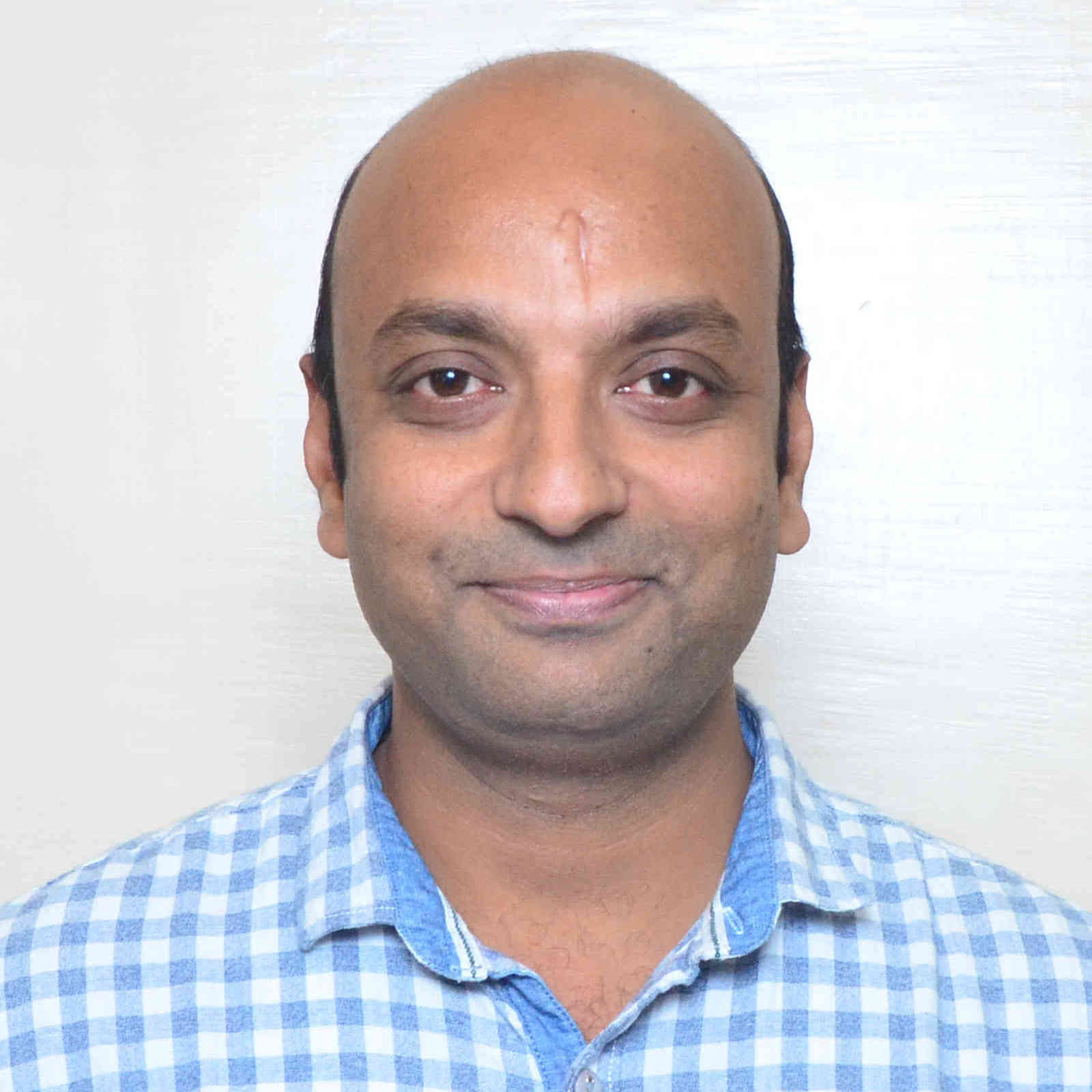Associate Professor
Interests :

Optimization theory, Duality, Support Vector Machines, Fuzzy mathematical programming,

s.gupta@ma.iitr.ac.in+91-1332-285379Associate Professor
Interests :

Computer Communications Networks, Performance Prediction of Wireless Systems, Networking, , Operations Research, Stochastic Processes, Queueing theory, Inventory Models, Software and hardware , Industrial Mathematics, Mathematical Modelling, Biomathematics,Associate Professor
Interests :

Numerical Analysis, Numerical Analysis and Simulations of PDEs, Wavelets Analysis, Finite Element Methods, Computational Modeling,

ram.jiwari@ma.iitr.ac.in8191957249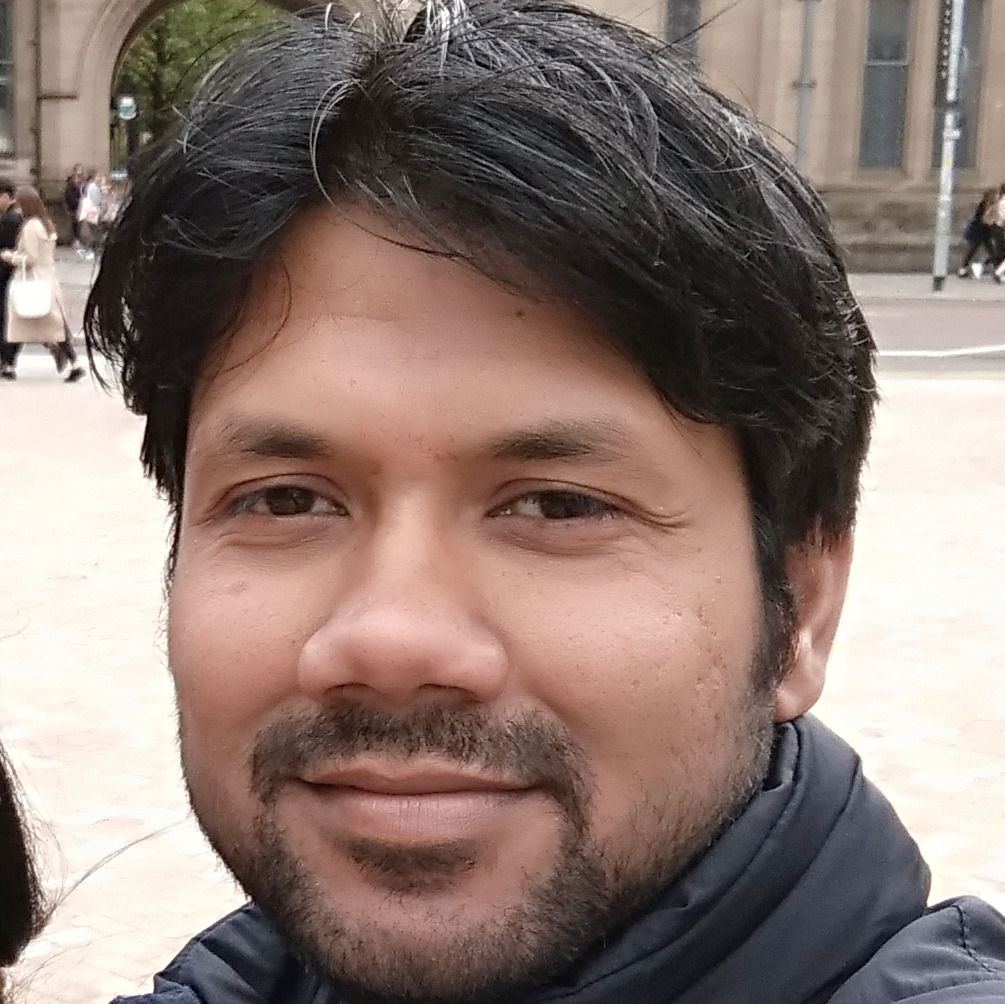Assistant Professor
Interests :

Numerical Solution of Partial Differential Equations, h-p finite element/Spectral Element Methods, Least Squares Method, Discontinuous Galerkin (DG) FEM, HDG FEM, A Posteriori Error Analysis, Multigrid Method, Parallel Computing, Domain Decomposition, Scientific Computing, Mixed FEM, Eigenvalue problems, Numerical Linear Algebra, Uncertainty Quantification, Stochastic Partial Differential Equation, Adaptivity,

arbaz@ma.iitr.ac.inAssistant Professor
Interests :

Probability and Stochastic Analysis:, MFG, Mckean-Vlasov equation, SDE, Levy Process, financial mathematics, stochastic gradient method,

c.kumarfma@iitr.ac.inAssociate Professor
Interests :

MATHEMATICAL IMAGE PROCESSING (Computational Algorithms for Image Restoration; Image Encryption and Secret Sharing; Quantum Imaging; 3-D Reconstruction), MACHINE LEARNING (Neural Networks and Tree-based Classifiers; Applications in Image Processing),

sanjeev.kumar@ma.iitr.ac.in01332-285824Associate Professor
Interests :

Coding Theory, Codes over rings, Locally recoverable codes, Boolean functions in coding theory and cryptography,

maheshanand@ma.iitr.ac.in01332-285543Assistant Professor
Interests :

Operator Theory; Functional Analysis,

amit.maji@ma.iitr.ac.in+91-1332-285392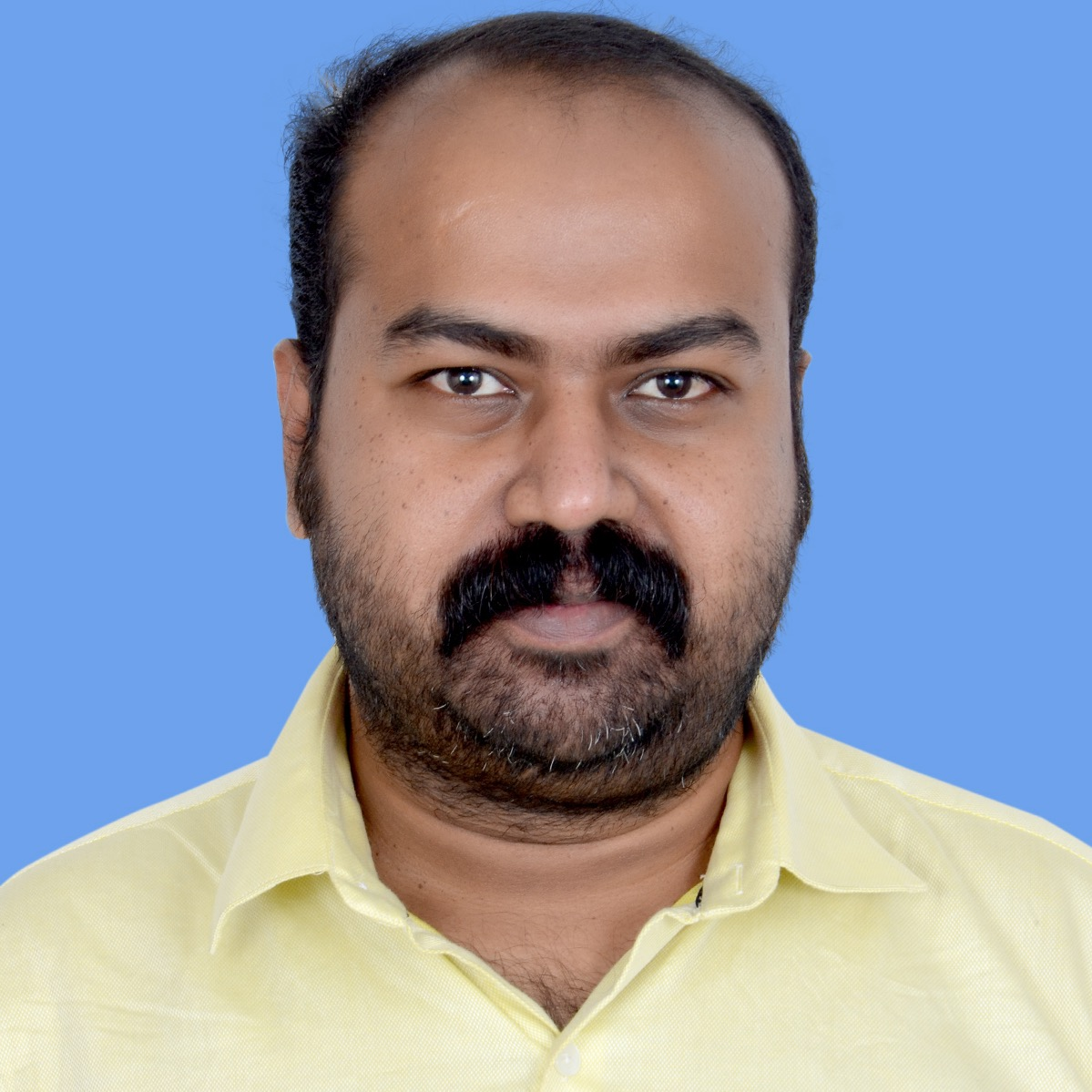Assistant Professor
Interests :

Stochastic (Partial) Differential Equations, Solvability, Large Deviation Theory, Ergodicity, Random Attractors, Stochastic Stability, Partial Differential Equations , Solvability and Stability of Parabolic and Hyperbolic Systems, Inverse Problems, Control Theory , Deterministic and Stochastic Control Theory in Infinite Dimensions, Optimal Control Theory, Mathematical Fluid Dynamics , Stochastic Analysis and Control of Incompressible and Compressible Fluids, Optimization, Problems with Orthogonal Matrix Constraints,

maniltmohan@ma.iitr.ac.in911332285319Associate Professor
Interests :

Computational Biophysics, Reactor Modelling, Finite element method, Population Balance Method., Fluid Dynamics, Electroosmotic Flows, Microfluidics, Two phase flow in porous media, Ventilation problems,

ameeya.nayak@ma.iitr.ac.in+91-1332-284777Associate Professor
Interests :

Differential equation, Functional Evolution Equation, Control theory, Semi-group theory, Fractional Differential Equations,

dwijendra.pandey@ma.iitr.ac.in01332-28-5735Associate Professor
Interests :

Number Theory (Additive, Combinatorial, and Elementary),

ram.pandey@ma.iitr.ac.in01332-284796Associate Professor
Interests :

Mob.No.+919897030710, Bio Mathematics, Computer Appl, Computational Fluid Dynamics, Lung Mechanics,

pratibha@ma.iitr.ac.inAssociate Professor
Interests :

Real Analysis, Complex Analysis, Fourier Approximation, Summability,Professor
Interests :

Computerized Tomography, Reconstruction Error Analysis, Convolution Backprojection, Image Processing,

tanuja.srivastava@ma.iitr.ac.in01332-285084Professor
Interests :

Nonlinear Analysis, Solvability of operator equations, Inverse problems, Control Theory, Controllability, stability and stabilizability, Fractional order systems, Robotics and Control, Trajectory tracking controller, Biped Robot Control, ANN based control,

n.sukavanam@ma.iitr.ac.in+911332285341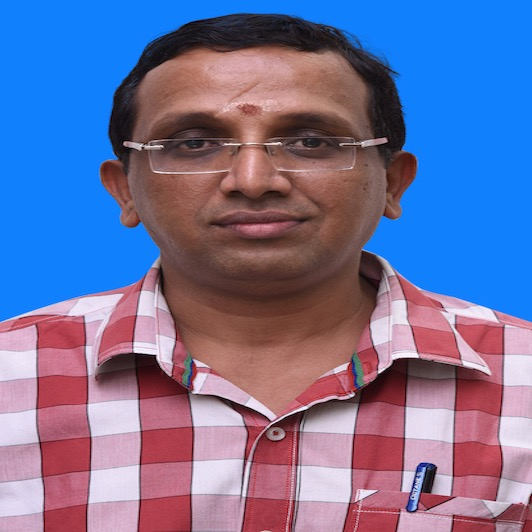Professor
Interests :

Complex Analysis, Orthogonal Polynomials and Special Functions, Functional Analysis: Operator Theory and Approximation Theory,

a.swaminathan@ma.iitr.ac.in01332-285182Assistant Professor
Interests :

Number Theory, Automorphic representations,

mahendraverma@ma.iitr.ac.in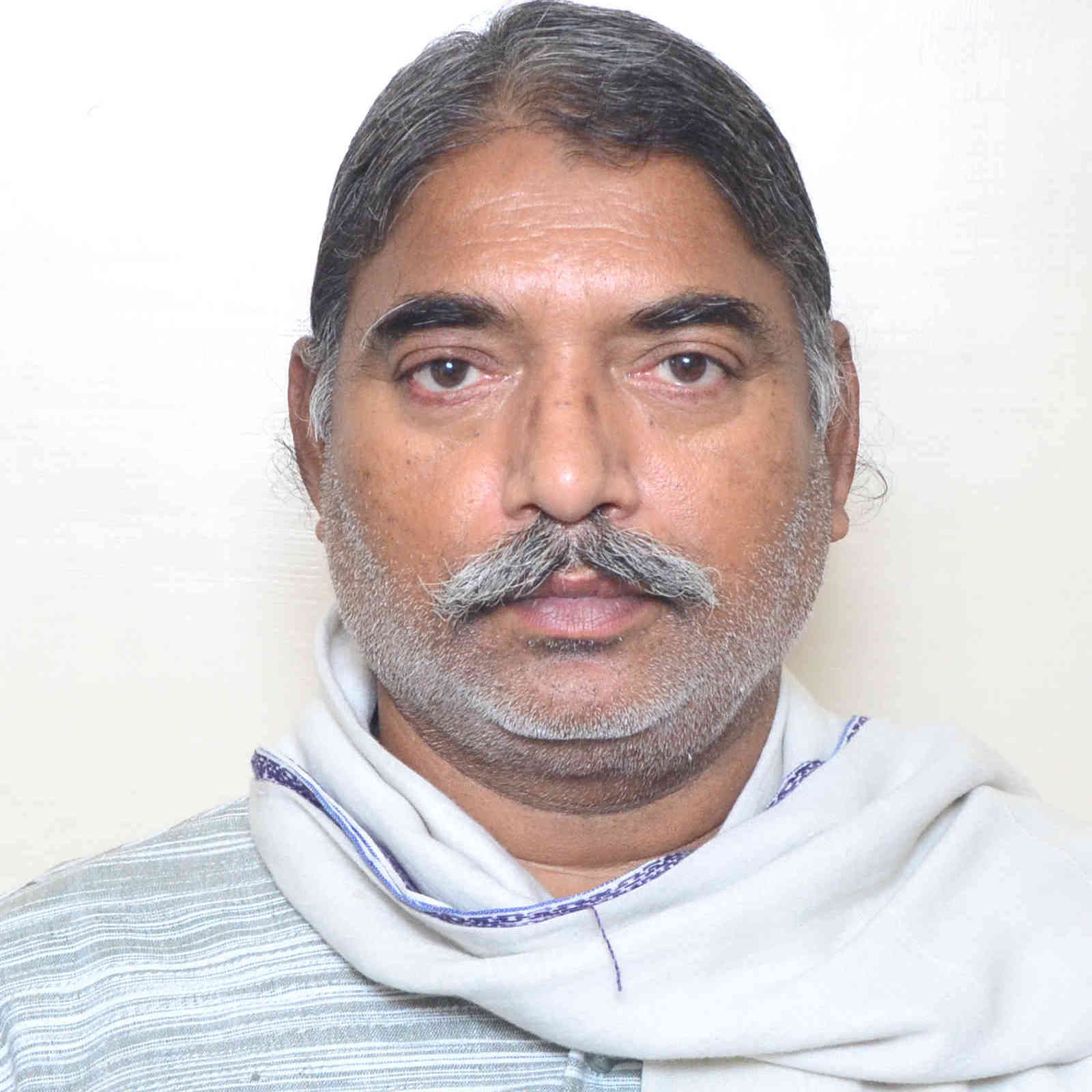Professor
Interests :

Operations Research, Optimal Control Theory, Fuzzy Maths, Intuitionistic Fuzzy Maths, Data Envelopement Analysis,